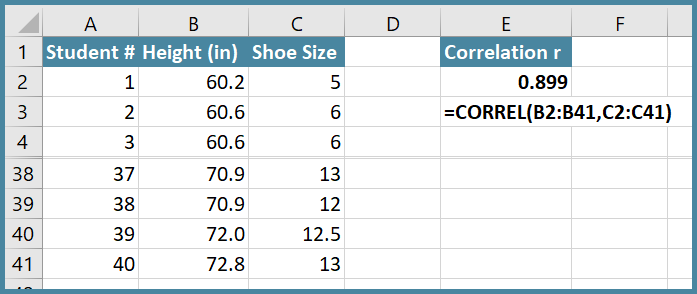# Correlation: Introduction to Relationships

In the last lesson, you learned about the Two-way Chi-square Test for Independence. Using it, you can determine if two categorical variables are independent. If two variables are not independent, they are related. Knowing something about one variable can tell you something about the other variable.

In this lesson, you will explore further this idea of variables being related, not being independent. Categorical variables that are related do not have a linear relationship for a number of reasons. One primary reason is that categorical variables are discrete, not continuous. This means that values of the categorical variable “jump” from one level to another rather than smoothly changing.

For example, if a survey has captured a person’s Age into buckets with 10-year intervals [e.g. 10 to 20, 21 to 30, 31 to 40,…], Age becomes a discrete categorical variable instead of being a continuous variable. If our Two-way Chi-square Test for Independence between Age and Gender in the survey data is statistically significant, we can just conclude that the variables Age and Gender are related. But we cannot as easily predict a survey participant’s Age given their Gender.

If you are considering two continuous variables, you can use their linear relationship, their correlation, to develop an equation you can use to predict values of one given a value of the other. You can use linear regression to do that. In this lesson, you will learn about linear regression using both one and multiple predictor variables to develop an equation you can use to estimate the value of the continuous response variable.

And although its predictions are a bit more complicated, you can use logistic regression to predict values of categorical response variables.

Correlation

Consider the following data table which shows a sample of 40 teenagers’ height in inches and American shoe size:Is there a relationship between Height and Shoe Size? I think common sense would suggest Yes, but how can you test that there is a relationship that can be used to predict?

You should always start data analysis by graphing the data. Here is a scatter chart (also known as x-y chart) created using Excel of these data:Using Excel to plot the data, you can easily add in a trend line which is a “best fit” line. There is an obvious pattern which indicates Shoe Size increases as Height increases.

This relationship can be quantified by calculating the correlation between the two variables. The sample correlation coefficient, r, can be quantified using the Excel function CORREL as shown in this image:

Note several rows of data (rows 5 through 37) have been hidden.The sample correlation coefficient, r, is 0.899. [An R is used to indicate the population correlation coefficient.]

The correlation coefficient can be negative, indicating the y-variable decreases as the x-variable increases. Or positive, indicating the y-variable increases as the x-variable increases. The largest negative value r can be is -1. The largest positive value r can be is +1. An r of 0 indicates no correlation.

The following graphs which illustrate the range of values r can take on were created using random numbers to generate x-y pairs which have the indicated correlation coefficient r.Alt-text: six scatter plots showing different correlation coefficients, ranging from absolute value 0.06 to 0.98. Generally, the closer the data points are to the trend line, the strong the correlation and larger r becomes.

Original image by D. Wright

Although the sign of r tells us if the slope of the trend line is positive (up) or negative (down), it is important to realize that the correlation coefficient r is not the mathematical slope of the trend line. The correlation coefficient r tells us the strength of the relationship of the two variables. In general, the closer the data points are to the trend line, the stronger the correlation.

Strength of Correlation

The strength the correlation is equal to the absolute value of the correlation coefficient r. The following table gives you a way to think of the strength of the correlation between two variables based on the absolute value of r.Correlation does not prove Causation

Regardless of the strength of the correlation, correlation alone does not prove causation. Remember there may be other factors at play that are causing the two variables to move together. This image is from a fun website called Spurious Correlations [http://www.tylervigen.com/spurious-correlations] created by Tyler Vigen. This is one plot on that site:Alt-text: x-y chart showing plots of data for the divorce rate in Maine each year from 2000 to 2009 and also the per capita margarine consumption in pounds per person.

Image adapted from tylervigen,com Spurious correlations.[ Copyleft notice: You are free to copy and adapt everything on this site. tylervigen.com] (Vigen, n.d)

Even though the r is large, 0.993, it should be obvious that there is no causative relationship between the Divorce Rate in Maine and the US per capita consumption of margarine. Showing causation requires more than just a correlation. See Hill’s Criteria Causation for more information (Fedak, Bernal, Capshaw, & Gross, 2015) [ https://dx.doi.org/10.1186%2Fs12982-015-0037-4 ] 20 min

Getting the equation of the trend line

Rather than calculate the correlation coefficient directly using the method shown above, you can get it by running a linear regression which will calculate and test the linear correlation between two variables, and also determine the equation of the trend line which can be used to predict values of y given a value of x. The regression will calculate the slope of the trend line and the y-Intercept which are needed for the equation of the line.

For a great visual explanation of correlation, check out this Stat Quest Video (https://youtu.be/xZ_z8KWkhXE )  19 min. to reinforce your understanding.

# References

Fedak, K., Bernal, A., Capshaw, Z., & Gross, S. (2015). Applying the Bradford Hill criteria in the 21st century: how data integration has changed causal inference in molecular epidemiology. Emerging Themes in Epidemiollogy, 12:14. doi:https://dx.doi.org/10.1186%2Fs12982-015-0037-4